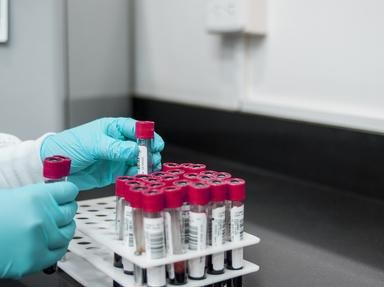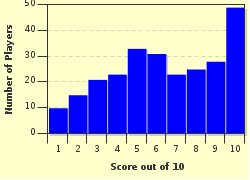# Mass Spectrometry Trivia Quiz

### Mass spectrometry is used to determine the structural formula of an unknown chemical compound. Its spectra reveal useful information such as the molecular weight and the elements present in the compound other than the typical C and H atoms.

A multiple-choice quiz by Matthew_07. Estimated time: 4 mins.

Author
Matthew_07
Time
4 mins
Type
Multiple Choice
Quiz #
295,815
Updated
Dec 03 21
# Qns
10
Difficulty
Average
Avg Score
6 / 10
Plays
1041
Last 3 plays: Guest 213 (0/10), Guest 116 (9/10), Guest 175 (5/10).
This quiz has 2 formats: you can play it as a or as shown below.
Scroll down to the bottom for the answer key.
1. Who was credited for the invention of the mass spectrometer? Hint

Ernest Rutherford
Neils Bohr
J.J. Thompson

#### NEXT>

2. In a mass spectrometer, molecules are bombarded with high-speed electrons and are ionized before broken into smaller fragments, namely cations and radicals. Which species will be detected by the mass spectrometer? Hint

None of these
Both of these
Cations

#### NEXT>

3. Ions formed in a mass spectrometer will be separated by magnetic deflection. The radius of curvature of the ion's path depends on the ratio of m/z. (m is the mass and z is the charge of the ion). Which of the following ions will bend the most? Hint

Ion D with m = 20 and z = +2
Ion B with m = 20 and z = +1
Ion C with m = 10 and z = +2
Ion A with m = 10 and z = +1

#### NEXT>

4. The peaks shown by a mass spectrum are given in terms of relative abundance, where the strongest peak (also known as the base peak) is assigned a value of 100%. On the other hand, the parent peak (also known as the molecular ion peak) is the peak shown by the molecular ion, M+ after losing only 1 electron (without undergoing any fragmentation). Will this parent peak always be the base peak?

Yes
No

#### NEXT>

5. The peak with the greatest m/z value for alcohols is given by m/z M-18, where M represents the molecular weight of the alcohols. The number 18 in "M-18" means that the radical cation loses a (an) ___ molecule. Hint

Carbon dioxide, CO2
Carbon monoxide, CO
Ethene, C2H4
Water, H2O

#### NEXT>

6. The most common fragmentation pattern for alkanes is the one that occurs at the molecules' branches.

True
False

#### NEXT>

7. The presence of which element in any compound is characterized by an odd number of m/z for its parent peak? Hint

Nitrogen, N
Oxygen, O
Sulfur, S
Phosphorus, P

#### NEXT>

8. You notice that a mass spectrum shows the equal intensity (peak) for both m/z at M+ (molecular ion) and M+2 (the molecular weight of the compound plus 2). Which element is present in the compound? Hint

Chlorine, Cl
Boron, B
Nitrogen, N
Bromine, Br

#### NEXT>

9. The mass spectrum of 2, 2-dimethylpropane, (CH3)4C shows a base peak (the peak with the highest intensity) at m/z 57. This m/z 57 corresponds to the (CH3)3C+ species. What kind of carbocation is it? Hint

Quaternary
Primary
Tertiary
Secondary

#### NEXT>

10. An unknown chemical compound is given to you and you run a test by using a normal mass spectrometer. From the spectrum, you know that the molecular weight of the compound is 28. This 28 indicates that your compound might be CO, C2H4 or N2, since the molecular formulae for these three compounds are 28 as well. You decide to put the compound in an HR-MS this time to determine the correct molecular formula of this compound. What does HR-MS stand for? Hint

High-Resolution Mass Spectrometer
Half-Resolution Mass Spectrometer
High-Resonance Mass Spectrometer
Half-Resonance Mass Spectrometer

 (Optional) Create a Free FunTrivia ID to save the points you are about to earn:Select a User ID:Choose a Password:Your Email:

Quiz Answer Key and Fun Facts
1. Who was credited for the invention of the mass spectrometer?

Joseph John Thompson was a British physicist. He also discovered the electron, which is one of the subatomic particles in an atom. He won the 1906 Nobel Prize in Physics for this discovery. He was the first person to measure the mass-to-charge ratio (m/z) of an electron.
2. In a mass spectrometer, molecules are bombarded with high-speed electrons and are ionized before broken into smaller fragments, namely cations and radicals. Which species will be detected by the mass spectrometer?

An electron will collide with a molecule, M. The resulting species are two electrons and the molecular/parent ion, M+. This M+ species is formed after one of its electrons is lost to the surrounding. It can be regarded as a cation radical before it is further ionized into a cation and a radical. A radical is a neutral species, as opposed to a positively charged cation.
3. Ions formed in a mass spectrometer will be separated by magnetic deflection. The radius of curvature of the ion's path depends on the ratio of m/z. (m is the mass and z is the charge of the ion). Which of the following ions will bend the most?

Answer: Ion C with m = 10 and z = +2

The radius of curvature is a measure of the degree of "bending" of a curve. Its symbol is kappa. A small circle would have a large curvature while a large circle would have a small curvature; a straight line's curvature is zero.

The degree of bending of an ion is inversely proportional to m (heavier ions bend less because of their inertia) but directly proportional to z (more positively charged ions will bend more). So in this case ion C will bend the most while ion B will bend the least.
4. The peaks shown by a mass spectrum are given in terms of relative abundance, where the strongest peak (also known as the base peak) is assigned a value of 100%. On the other hand, the parent peak (also known as the molecular ion peak) is the peak shown by the molecular ion, M+ after losing only 1 electron (without undergoing any fragmentation). Will this parent peak always be the base peak?

No, the base peak actually comes from the structure with the highest stability, that is, the one that shows resonance.

For example, the compound dimethtyl ether, CH3-O-CH3 will have the parent peak at m/z 46 (which also corresponds to its molecular weight, 46 atomic mass units). However, its base peak is at m/z 31, which comes from the CH2=O+H stabilized cation species.

For molecules that show no resonance structures, their parent peak might be the base peak as well.
5. The peak with the greatest m/z value for alcohols is given by m/z M-18, where M represents the molecular weight of the alcohols. The number 18 in "M-18" means that the radical cation loses a (an) ___ molecule.

Notice that the molecular weight for water, H20 is 18.

Normally, a radical cation may lose small molecules, such as H20, CO, CO2 and alkenes (ethane, propene, etc).

In the case of alcohols, the most common case is the loss of a water molecule, which corresponds to the M-18 peak.
6. The most common fragmentation pattern for alkanes is the one that occurs at the molecules' branches.

Fragmentation at the molecules' branches will yield carbocations with higher stability.
7. The presence of which element in any compound is characterized by an odd number of m/z for its parent peak?

Any chemical compound that has 1 N atom in it will have an odd value for its molecular formula. For example, the molecular formula for CH3NH2 is 31.
8. You notice that a mass spectrum shows the equal intensity (peak) for both m/z at M+ (molecular ion) and M+2 (the molecular weight of the compound plus 2). Which element is present in the compound?

The bromine atoms occur naturally in two isotopes, namely Br-79 and Br-81. Their ratio is approximately 1:1. So, if a compound contains a Br atom, the peak at M+ and M+2 would show the same intensity or height.

On the contrary, the M+2's peak of a compound with a Cl atom in it would be a third as large as the M+2's peak. This is because the ratio of the two Cl isotopes, namely C1-35 and Cl-37 is 24.5:75.5 which is close to 1:3.
9. The mass spectrum of 2, 2-dimethylpropane, (CH3)4C shows a base peak (the peak with the highest intensity) at m/z 57. This m/z 57 corresponds to the (CH3)3C+ species. What kind of carbocation is it?

The general formulae for the primary, secondary and tertiary carbocations are (R)(R')(R')C+, (R)(R')CH+ and RCH2+ respectively. The alkyl groups in parentheses indicate they are attached to the carbon atom that bears the positive charge. There is no such thing as quaternary carbocation.

A tertiary carbocation has the highest stability, followed by a secondary one and a primary one.
10. An unknown chemical compound is given to you and you run a test by using a normal mass spectrometer. From the spectrum, you know that the molecular weight of the compound is 28. This 28 indicates that your compound might be CO, C2H4 or N2, since the molecular formulae for these three compounds are 28 as well. You decide to put the compound in an HR-MS this time to determine the correct molecular formula of this compound. What does HR-MS stand for?

An HR-MS (High-Resolution Mass Spectrometer) can detect the mass of the molecule to an accuracy of 5 decimal places. The exact molecular weights of CO, C2H4 and N2 are 27.99491, 28.03130 and 28.00610 respectively. By comparing the molecular weight determined by a HR-MS, you can easily compare the value obtained with the three values above.
Source: Author Matthew_07

This quiz was reviewed by FunTrivia editor crisw before going online.
Any errors found in FunTrivia content are routinely corrected through our feedback system.
Most Recent Scores
Feb 01 2023 : Guest 213: 0/10
Jan 31 2023 : Guest 116: 9/10
Jan 31 2023 : Guest 175: 5/10
Jan 30 2023 : Guest 49: 1/10
Jan 30 2023 : Guest 37: 3/10
Jan 30 2023 : cdecrj: 2/10
Jan 30 2023 : Guest 94: 3/10
Jan 30 2023 : Guest 68: 6/10
Jan 20 2023 : Guest 131: 10/10

Score DistributionRelated Quizzes
This quiz is part of series Matthew's Chemistry Quizzes:

A collection of my chemistry quizzes encompassing the disciplines of organic and analytical chemistry. This list covers topics on elements, chemical functional groups, and specialized analytical techniques.

1. Hydrogen - The Lightest Element Average
2. Helium - The Balloon Gas Average
3. Gold, Silver and Bronze Average
4. Matter and Substance Average
5. Elementary Scientists and Mathematicians Average
6. Ethers and Epoxides Tough
7. Analytical Chemistry Tough
8. Infrared Spectroscopy Average
9. Mass Spectrometry Average